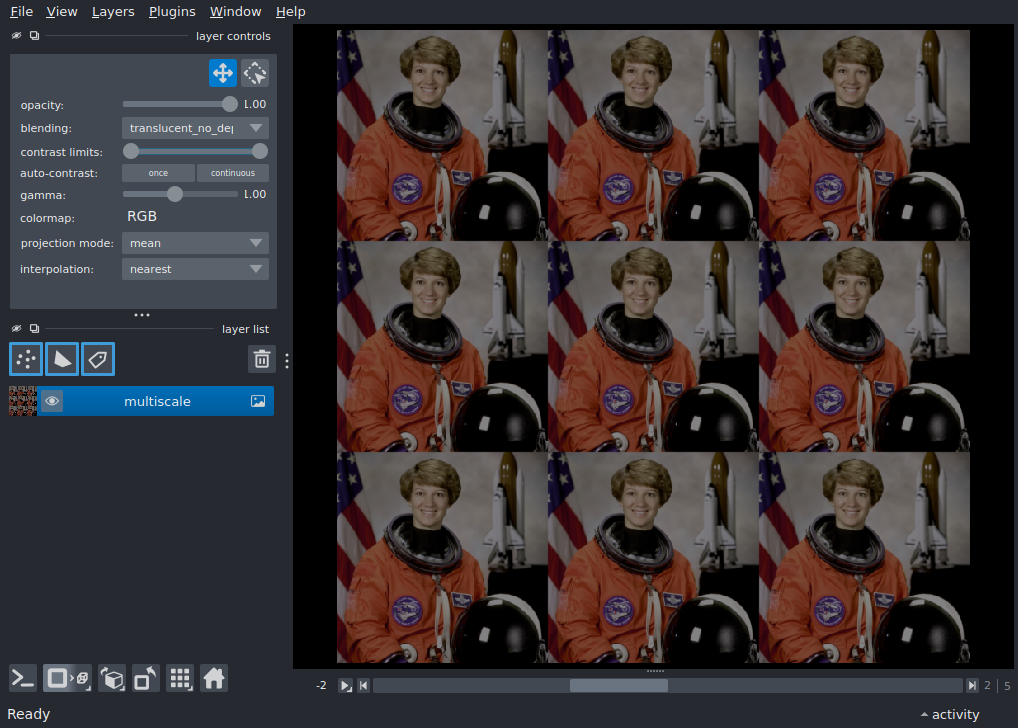# nD multiscale image non-uniform¶

Displays an nD multiscale image```/home/runner/work/napari/napari/examples/nD_multiscale_image_non_uniform.py:20: FutureWarning: `multichannel` is a deprecated argument name for `pyramid_gaussian`. It will be removed in version 1.0. Please use `channel_axis` instead.
pyramid_gaussian(base, downscale=2, max_layer=3, multichannel=True)
multiscale level shapes:  [(6, 1536, 1536, 3), (6, 768, 768, 3), (6, 384, 384, 3), (6, 192, 192, 3)]
```

```from skimage import data
from skimage.transform import pyramid_gaussian
import napari
import numpy as np

# create multiscale from astronaut image
astronaut = data.astronaut()
base = np.tile(astronaut, (3, 3, 1))
multiscale = list(
pyramid_gaussian(base, downscale=2, max_layer=3, multichannel=True)
)
multiscale = [
np.array([p * (abs(3 - i) + 1) / 4 for i in range(6)]) for p in multiscale
]
print('multiscale level shapes: ', [p.shape for p in multiscale])